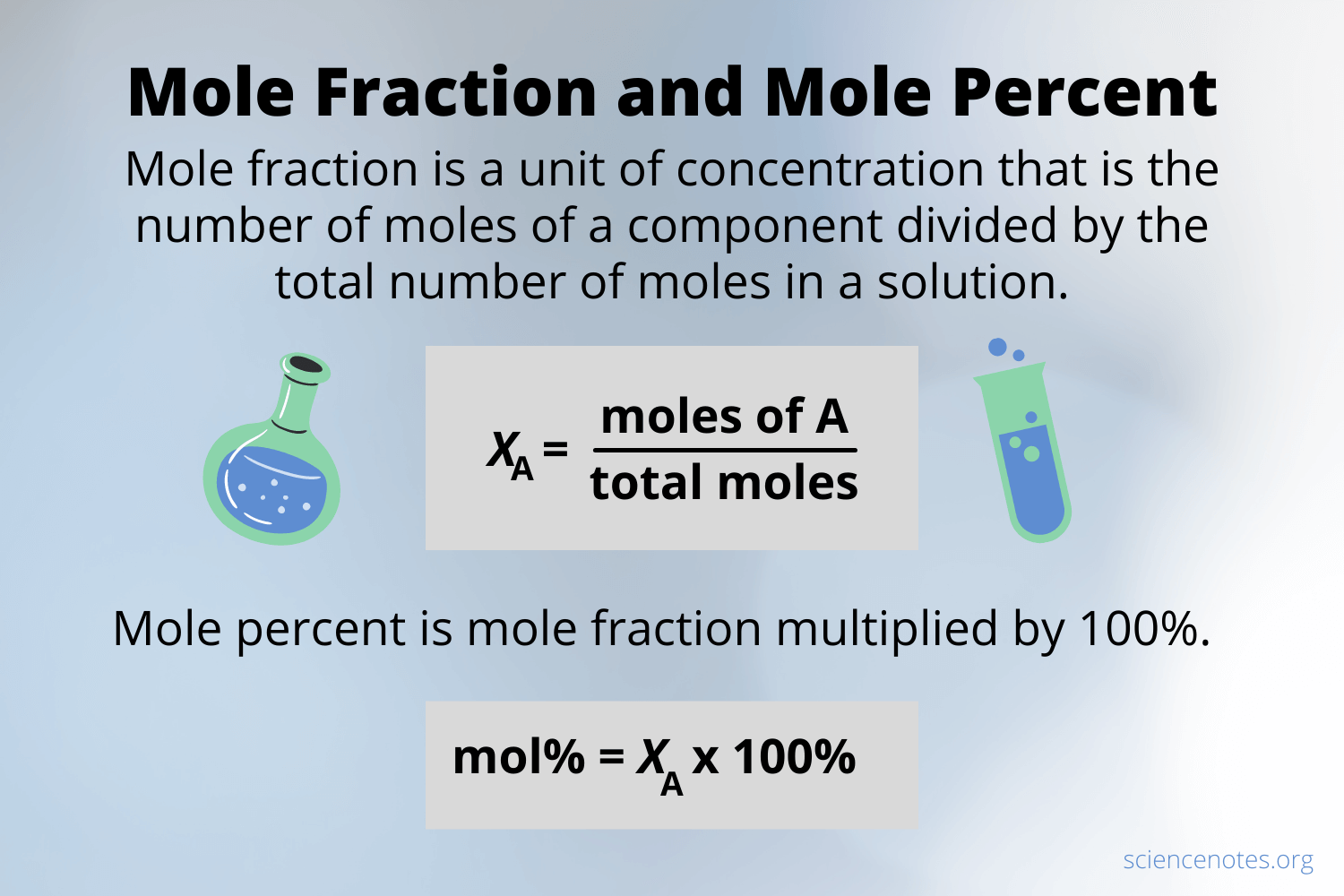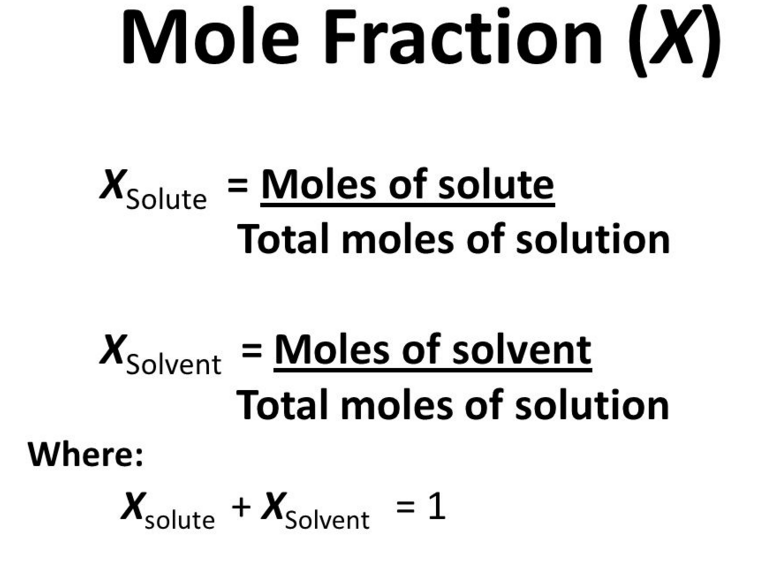HS-PS1-5

# Mole Fraction Study Guide

Calculating the concentration of elements in a reaction can be understood using mole fraction.

## INTRODUCTION

There is nothing as satisfying as perfectly cooked pasta. Everyone knows what ingredients are used for cooking pasta, but is it enough? The perfection of the dish lies in the concentration (what and how much) of the ingredients used. From the beginnings of chemistry to the most complex methods, concentration and its consequences are essential.Source

Concentration is crucial from a conceptual standpoint. It informs you how much you possess and what quantity you have. Without the right concentrations, a chemical reaction that requires specific amounts of reagents may simply not function. Concentration is directly related to determining information such as the amount of heavy metal in freshwater, the amount of insecticide that has run off into a neighboring water source, and the severity of acid rain.

### WHAT IS THE MOLE FRACTION?

The mole fraction is one of the numerous methods for indicating the concentration of a solution. A mole fraction is a concentration unit. The mole fraction, symbolized by "X," determines the relative concentration of solutes and solvents in a solution.

For a mixture of two substances, C and D, the mole fraction equation of each would be written as follows:

Xc = mol C / mol C + mol D

Xd = mol D / mol C + mol D

Mole fractions have no dimensions hence the total of all mole fractions in a combination is always 1.Source

### MOLE FRACTION EXAMPLE

If 0.010 moles of NaCl are dissolved in 100 grams of pure water, compute the mole fraction of NaCl and H₂O.

We know, the molecular weight of water is 18.0153

• Number of moles of water = 100/18.015= 5.56 moles
• Mole fraction of NaCl = 0.10/ 5.56 + 0.100 = 0.018
• Mole fraction of H₂O = 5.56/5.66 = 0.982
• Sum of mole fractions here is 0.982 + 0.018 = 1

In conjunction with Dalton's law of partial pressures, a mole fraction is a helpful number for studying gas mixtures. Think about the following scenario:

A 1.5 atm total pressure is exerted on the container walls by hydrogen and oxygen gas mixture. Find the partial pressure of oxygen and hydrogen in the mixture if the mole fraction of hydrogen is 0.67.

Make a list of the known quantities and devise a strategy for solving the problem.

• Total pressure = 1.5 atm

• Mole fraction of oxygen = 0.33

• Mole fraction of hydrogen = 0.67 Unknown

• The partial pressure of oxygen (Po)

• The partial pressure of hydrogen (Ph)

• Now, multiplying the mole fraction by the total pressure yields the partial pressure.

• Ph = 0.67 × 1.5 = 1 atm and Po = 0.33 × 1.5 = 0.5 atm

### PROPERTIES OF MOLE FRACTION

• It is not necessary to know the densities of the states of the elements involved.
• The mole fraction of an ideal gas mixture can be calculated as the ratio of partial pressure to total pressure. This scale is symmetrical.
• A mixture of known mole fractions can be created by measuring the right masses of the elements.

## CONCLUSION:

• A mole fraction is a concentration unit symbolized by "X."

• Mole fractions have no dimensions hence the total of all mole fractions in a combination is always 1.

• For a mixture of two substances, C and D, the mole fraction equation of each would be written as follows:

``````                   Xc = mol C / mol C + mol D

Xd = mol D / mol C + mol D
``````

### FAQs:

1. What is the mole fraction formula?

The formula of mole fraction is the ratio of the moles of solute/ solvent to the total moles of solute and solvent.

2. What is meant by mole fraction?

Mole fraction is a concentration unit that determines the relative concentration of solutes and solvents in a solution.

We hope you enjoyed studying this lesson and learned something cool about the Mole Fraction! Join our Discord community to get any questions you may have answered and to engage with other students just like you! Don't forget to download our App to experience our fun VR classrooms - we promise, it makes studying much more fun! 😎

## SOURCES:

1. Mole Fraction. https://www.ck12.org/c/chemistry/mole-fraction/lesson/Mole-Fraction-CHEM/. Accessed 16 Feb 2022.
2. Concentration Units. https://courses.lumenlearning.com/trident-boundless-chemistry/chapter/concentration-units/. Accessed 16 Feb 2022.